# ISEE Middle Level Math : Algebraic Concepts

## Example Questions

← Previous 1 3 4 5 6 7 8 9 61 62

### Example Question #1 : Variables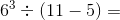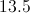Explanation:

First find the exponent value: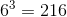Then find the value of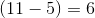Finally, solve the entire expression with the known values: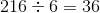### Example Question #31 : Algebra

Simplify: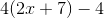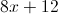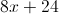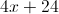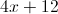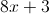Explanation: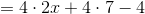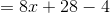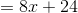### Example Question #6 : Multiplying And Dividing Polynomials

Multiply: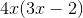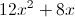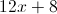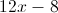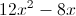Explanation:

Use the distributive property: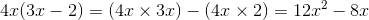### Example Question #3 : Variables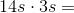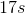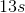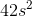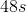Explanation:

Multiply the numbers and multiply the variables: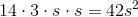Answer:### Example Question #1 : Algebraic Concepts

Simplify: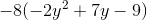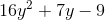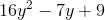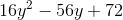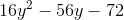Explanation: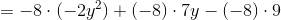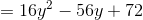### Example Question #1 : Algebraic Concepts

Multiply: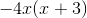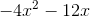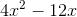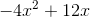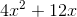Explanation: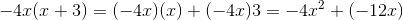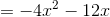### Example Question #1 : Algebraic Concepts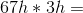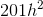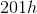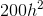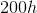Explanation:

Multiply the whole numbers and add an exponent to the variable totaling the number of exponents in the equation: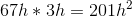Answer:### Example Question #7 : Variables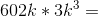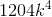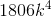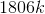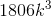Explanation:

Multiply the whole numbers and add an exponent to the variable totaling the number of exponents in the equation: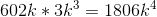Answer:### Example Question #8 : Variables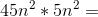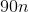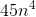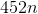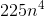Explanation:

Multiply the whole numbers and add an exponent to the variable totaling the number of exponents in the equation: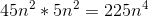Answer:### Example Question #9 : Variables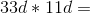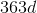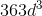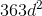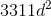Explanation:

Multiply the constants and add an exponent to the variable totaling the number of variables in the equation: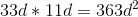Answer:← Previous 1 3 4 5 6 7 8 9 61 62

### All ISEE Middle Level Math Resources